# 深度学习部署-调用c++进行前向处理tensorflow1.4 c++编译以及API使用

###### tensorflow1.4 c++编译以及API使用

1. 安装bazel，然后使用bazel编译tensorflow源码，产生我们需要的库文件。
1. 在python环境下，使用tensorflow训练一个深度神经网络，本文以mnist为例。将训练好的模型和参数冻结在一个pb文件中。
1. 在C++环境下，调用pb文件，对图片进行预测。最终结果如下图所示，程序成功识别到图片中的数字为1，且概率为0.95。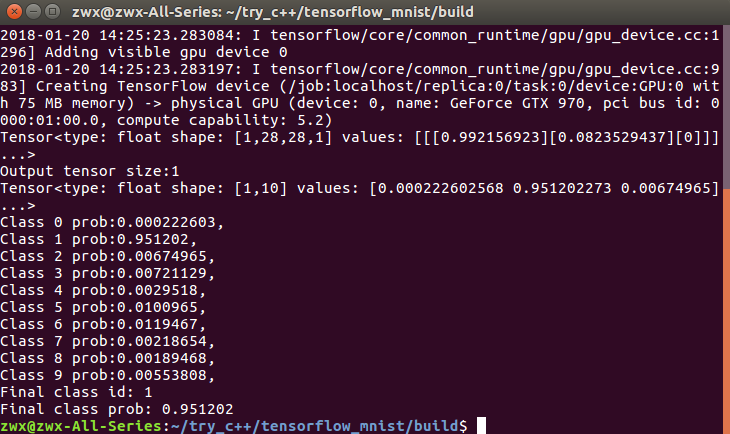https://github.com/zhangcliff/tensorflow-c-mnist.git

##### 1.安装bazel
``````echo "deb [arch=amd64] http://storage.googleapis.com/bazel-apt stable jdk1.8" | sudo tee /etc/apt/sources.list.d/bazel.list`
curl https://bazel.build/bazel-release.pub.gpg | sudo apt-key add -
sudo apt-get update
sudo apt-get install bazel
``````
##### 2.tensorflow的下载。

`git clone https://github.com/tensorflow/tensorflow.git`

##### 3.tensorflow的c++编译。

3.1 进入tensorflow文件夹中，首先进行项目配置。
`./configure`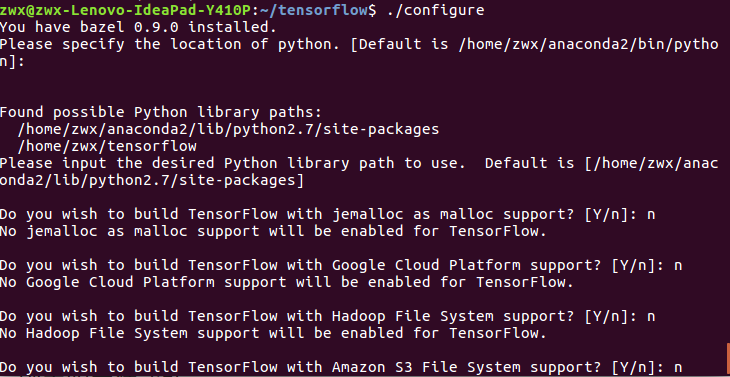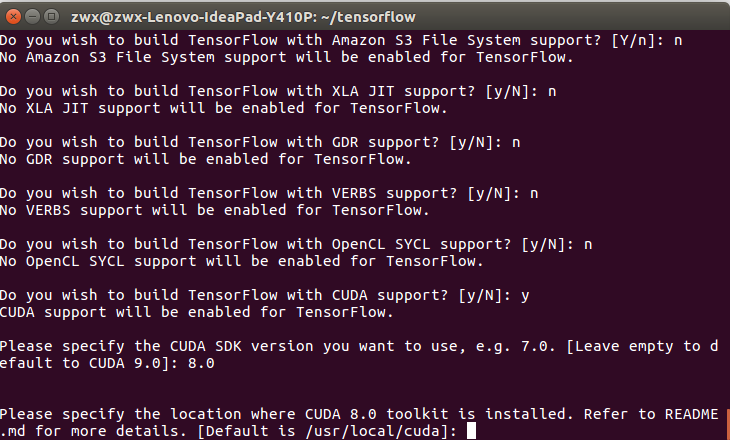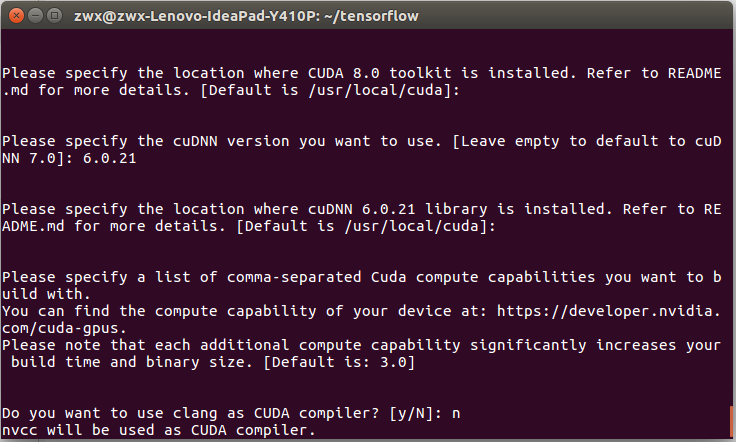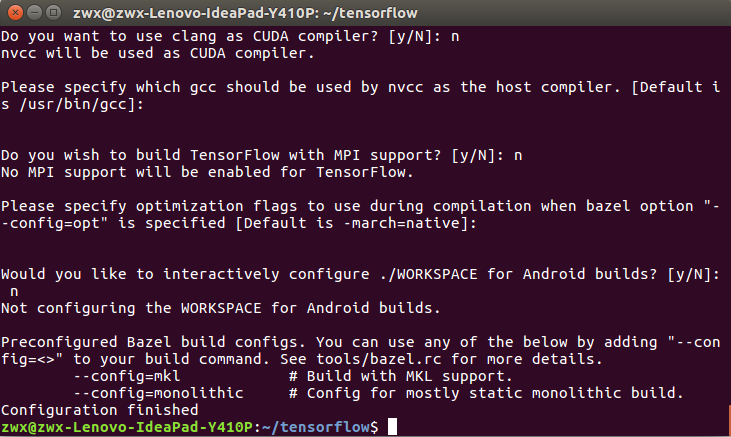3.2 使用bazel命令进行编译。编译的时间比较长，我在i3-4150cpu上编译了一个小时左右的时间。

``````bazel build --config=opt --config=cuda //tensorflow:libtensorflow_cc.so
``````

``````bazel build --config=opt //tensorflow:libtensorflow_cc.so
``````

##### 4. 使用tensorflow C++ api调用图模型（.pb文件）。

tensorflow 编译好之后，我们使用tensorflow c++ api调用一个已经冻结的图模型（.pb文件）

https://github.com/zhangcliff/tensorflow-c-mnist.git
4.1 在python环境下生成一个图模型（.pb文件）

``````from tensorflow.python.framework.graph_util import convert_variables_to_constants
graph = convert_variables_to_constants(sess, sess.graph_def, ["softmax"])
tf.train.write_graph(graph,'models','model.pb',as_text=False)
``````

write_graph()函数生成.pb文件，第二个参数为生成pb文件的文件夹，第三个参数为pb文件的名字。

4.2 c++环境下调用pb文件。

``````Session* session;
Status status = NewSession(SessionOptions(), &session);//创建新会话Session
string model_path="model.pb";
GraphDef graphdef; //Graph Definition for current model
std::cout << status_load.ToString() << " ";

return -1;
}
Status status_create = session->Create(graphdef); //将模型导入会话Session中;
if (!status_create.ok()) {
std::cout << "ERROR: Creating graph in session failed…" << status_create.ToString() << std::endl;
return -1;
}
cout << "Session successfully created."<< endl;
``````

``````Status ReadTensorFromImageFile(const string& file_name, const int input_height,
const int input_width, const float input_mean,
const float input_std,
std::vector<Tensor>* out_tensors)
``````

static Status ReadEntireFile(tensorflow::Env* env, const string& filename,
Tensor output)

``````const Tensor& resized_tensor = resized_tensors;
vector<tensorflow::Tensor> outputs;
string output_node = "softmax";
Status status_run = session->Run({{"inputs", resized_tensor}}, {output_node}, {}, &outputs);
``````

``````print(sess.graph.get_operations())
print(sess.graph.get_operations()）
print(sess.graph.get_operations())
``````

``````Tensor t = outputs; // Fetch the first tensor
auto tmap = t.tensor<float, 2>(); // Tensor Shape: [batch_size, target_class_num]
int output_dim = t.shape().dim_size(1); // Get the target_class_num from 1st dimension
// Argmax: Get Final Prediction Label and Probability
int output_class_id = -1;
double output_prob = 0.0;
for (int j = 0; j < output_dim; j++)
{
std::cout << "Class " << j << " prob:" << tmap(0, j) << "," << std::endl;
if (tmap(0, j) >= output_prob) {
output_class_id = j;
output_prob = tmap(0, j);
}
}
std::cout << "Final class id: " << output_class_id << std::endl;
std::cout << "Final class prob: " << output_prob << std::endl;
``````

4.3 使用cmake进行编译

``````cmake_minimum_required (VERSION 2.8.8)
project (tf_example)
set(CMAKE_CXX_FLAGS "\${CMAKE_CXX_FLAGS} -g -std=c++11 -W")
include_directories(
/home/zwx/tensorflow
/home/zwx/tensorflow/bazel-genfiles
/usr/local/include/eigen3
/home/zwx/tensorflow/bazel-bin/tensorflow
/home/zwx/tensorflow/tensorflow/contrib/makefile/gen/protobuf/include
)
``````

``````cd build
cmake ..
make
``````

`./tensorflow/contrib/makefile/download_dependencies.sh`

``````mkdir build
cd build
cmake ..
make
sudo make install
``````

4.4 运行可执行程序

make成功后，在build目录下会出现一个可执行文件tf_test。将一张28*28的数字图片也放在build路径下，文件名为digit.jpg，最后执行tf_test文件。

`./tf_test digit.jpg`

https://github.com/zhangcliff/tensorflow-c-mnist.git